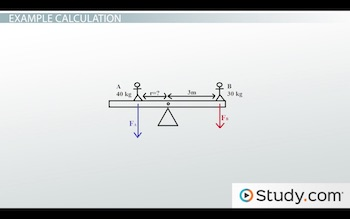# Torque: Concept, Equation & Example

An error occurred trying to load this video.

Try refreshing the page, or contact customer support.

Coming up next: Rotational Inertia & Change of Speed

### You're on a roll. Keep up the good work!

Replay
Your next lesson will play in 10 seconds
• 0:05 What is Torque?
• 1:16 Equation
• 2:07 Example Calculation
• 4:01 Lesson Summary

Want to watch this again later?

Timeline
Autoplay
Autoplay
Speed

#### Recommended Lessons and Courses for You

Lesson Transcript
Instructor: David Wood

David has taught Honors Physics, AP Physics, IB Physics and general science courses. He has a Masters in Education, and a Bachelors in Physics.

After watching this lesson, you will be able to explain what torque is conceptually and mathematically and use both the equation and Newton's First Law for rotation to solve problems involving torque. A short quiz will follow.

## What is Torque?

When you push hard enough on something, it moves. But it can move in lots of different ways. If you push straight down in the middle of an object, it will move forwards. But if you push off to one side, it might not. If you push a merry-go-round towards the center, nothing will happen. You have to push it in a direction that doesn't go towards the center.

A torque is just a force that is applied out of line with the center of mass of an object, a force applied a distance 'r' away from the center of mass, that causes the object to rotate. A bigger force means a bigger torque, and a larger distance from the pivot point means a bigger torque. And just like with linear forces, unbalanced torques can cause an acceleration - in this case, an angular acceleration. They cause the rate of rotation to change.

In everyday life, you have certain intuitions, things you've learned just by being in the world, by touching things, pushing things, and doing your own mini science experiments every day, perhaps to the irritation of your parents when you were a child. For example, you know that it will be harder to open a door if you push near the hinges. Instead, you push closer to the outside edge. By doing this, you increase the distance from the pivot point and increase the torque.

Levers also work by torque. A larger lever makes it easier to move heavy objects, because the distance from the pivot point is increased.

## Equation

We can take that intuitive, conceptual understanding and turn it into an equation. We know that the torque is proportional to the size of the force you use: bigger pushes will cause more of a change in the rotation. We also know that larger levers, which create larger perpendicular distances, will do the same. So torque must be proportional to both these things.

Mathematically, torque is the force, measured in newtons, multiplied by the distance to the pivot point from where the force is being applied, measured in meters. So torque as a number is measured in newton-meters.

Since torque is just a rotational version of force, we can also apply Newton's First Law to this equation. Similarly, for an object to be at rest or at a constant rate of rotation, the torques must be balanced. The clockwise torques must be equal to the counterclockwise torques. This is extremely useful for solving problems. So let's do an example.

## Example Calculation

Let's say you have a seesaw, and that seesaw has two friends sitting on each end. One weighs 40 kilograms, and the other weighs 30 kilograms. The one that weighs 40 moves closer to the middle, reducing the torque, until the seesaw is perfectly balanced. If the 30 kilogram person is 3 meters away from the pivot point, how far away from the pivot point is his friend?

Okay, so since the seesaw is balanced, we know that the clockwise torques must be equal to the counterclockwise torques.To unlock this lesson you must be a Study.com Member.

### Register to view this lesson

Are you a student or a teacher?

### Unlock Your Education

#### See for yourself why 30 million people use Study.com

##### Become a Study.com member and start learning now.
Back
What teachers are saying about Study.com

### Earning College Credit

Did you know… We have over 160 college courses that prepare you to earn credit by exam that is accepted by over 1,500 colleges and universities. You can test out of the first two years of college and save thousands off your degree. Anyone can earn credit-by-exam regardless of age or education level.